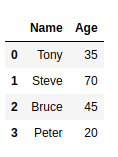Related Articles
How to Convert Pandas DataFrame into a List?
• Last Updated : 03 May, 2021

Let’s discuss how to convert Pandas dataframe to List. First, let’s create a Basic DataFrame:

## Python3

 `import` `pandas as pd ` `# Creating a dictionary to store data``data ``=` `{``'Name'``:[``'Tony'``, ``'Steve'``, ``'Bruce'``, ``'Peter'` `],``        ``'Age'``: [``35``, ``70``, ``45``, ``20``] } ` `# Creating DataFrame ``df ``=` `pd.DataFrame(data) ` `# Print the dataframe``df`

Output :At times, you may need to convert your pandas dataframe to List. To accomplish this task, ‘ tolist() ‘ function can be used. Below is a basic example to use this function and convert the required DataFrame into a List.

## Python3

 `df.values.tolist()`

Output :

`[['Tony', 35], ['Steve', 70], ['Bruce', 45], ['Peter', 20]]`

Here, Each inner list contains all the columns of a particular row.

Pandas DataFrame can be converted into lists in multiple ways. Let’s have a look at different ways of converting a DataFrame one by one.

Method #1: Converting a DataFrame to List containing all the rows of a particular column:

## Python3

 `import` `pandas as pd ` `# Creating a dictionary to store data``data ``=` `{``'Name'``:[``'Tony'``, ``'Steve'``, ``'Bruce'``, ``'Peter'` `] ,``        ``'Age'``: [``35``, ``70``, ``45``, ``20``] } ` `# Creating DataFrame ``df ``=` `pd.DataFrame(data) ` `# Converting DataFrame to a list containing``# all the rows of column 'Name'``names ``=` `df[``'Name'``].tolist()` `# Printing the converted list.``print``(names)`

Output:

`['Tony', 'Steve', 'Bruce', 'Peter']`

Method #2: Converting a DataFrame to Nested List containing all the rows of all the columns:

## Python3

 `import` `pandas as pd ` `# Creating a dictionary to store data``data ``=` `{``'Name'``:[``'Tony'``, ``'Steve'``, ``'Bruce'``, ``'Peter'` `] ,``        ``'Age'``: [``35``, ``70``, ``45``, ``20``] } ` `# Creating DataFrame``df ``=` `pd.DataFrame(data)` `# Creating an empty list``res``=``[]` `# Iterating through the columns of``# dataframe``for` `column ``in` `df.columns:``    ` `    ``# Storing the rows of a column``    ``# into a temporary list``    ``li ``=` `df[column].tolist()``    ` `    ``# appending the temporary list``    ``res.append(li)``    ` `# Printing the final list``print``(res)`

Output:

`[['Tony', 'Steve', 'Bruce', 'Peter'], [35, 70, 45, 20]]`

Method #3: Converting a DataFrame to a list that contains lists having all the columns of a row.

## Python3

 `import` `pandas as pd ` `# Creating a dictionary to store data``data ``=` `{``'Name'``:[``'Tony'``, ``'Steve'``, ``'Bruce'``, ``'Peter'` `] ,``        ``'Age'``: [``35``, ``70``, ``45``, ``20``] } ` `# Creating DataFrame``df ``=` `pd.DataFrame(data) ` `# Converting dataframe to list``li ``=` `df.values.tolist()` `# Printing list``print``(li)`

Output :

`[['Tony', 35], ['Steve', 70], ['Bruce', 45], ['Peter', 20]]`

Method #4: Converting a DataFrame to a list that contains lists having all the columns of a row along with column names.

## Python3

 `import` `pandas as pd ` `# Creating a dictionary to store data``data ``=` `{``'Name'``:[``'Tony'``, ``'Steve'``, ``'Bruce'``, ``'Peter'` `] ,``        ``'Age'``: [``35``, ``70``, ``45``, ``20``] } ` `# Creating DataFrame``df ``=` `pd.DataFrame(data) ` `# Converting dataframe to list``li ``=` `[df.columns.values.tolist()] ``+` `df.values.tolist()` `# Printing list``print``(li)`

Output:

[[‘Name’, ‘Age’], [‘Tony’, 35], [‘Steve’, 70], [‘Bruce’, 45], [‘Peter’, 20]]

Attention geek! Strengthen your foundations with the Python Programming Foundation Course and learn the basics.

To begin with, your interview preparations Enhance your Data Structures concepts with the Python DS Course. And to begin with your Machine Learning Journey, join the Machine Learning – Basic Level Course

My Personal Notes arrow_drop_up